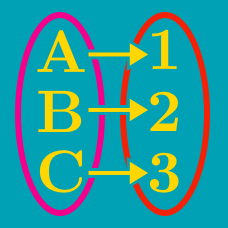Probability

# Injection and Surjection

Consider the two sets $X=\{1,2,3,4\}, Y=\{-8, 0, 20\}.$ Let $f$ be a surjective function from $X$ to $Y$ such that for any two elements $x_1$ and $x_2$ of $X,$ if $x_1 < x_2,$ then $f(x_1) \leq f(x_2).$ What is the minimum possible value of $f(4)$?

A function $f$ maps the elements of $A = \{14, 16, 18, 20\}$ to elements of $B =\{55, 66, 77, 88, 99\}.$ How many of the possible maps $f$ are not injective?

Details and assumptions

A function is injective if each element in the codomain is mapped onto by at most one element in the domain.

For two sets $X=\{a,b,c\}, Y=\{7, 11, 13, 17, 25, 32\},$ $f$ is an injective function from $X$ to $Y$. If $f(a)=7$ and $f(b)=17$, what is the sum of all the elements of $Y$ that can possibly be the value of $f(c)$?

For $X=\{-2,-1,0,1,2\},$ function $f$ is surjective from $X$ to $Y,$ where $f(x) = \begin{cases} x+4 & \text{ if } x > 0, \\ -x^3+18 & \text{ if } x \leq 0. \end{cases}$ What is the sum of all the elements of $Y?$

For two sets $X=\{a,b,c\}, Y=\{y\mid 1 \leq y \leq 7, y \text{ is an integer}\},$ how many injective functions $f: X \to Y$ exist?

×